CBSE Class 10 Sample Paper for 2022 Boards - Maths Standard [MCQ]

Class 10
Solutions of Sample Papers for Class 10 Boards

##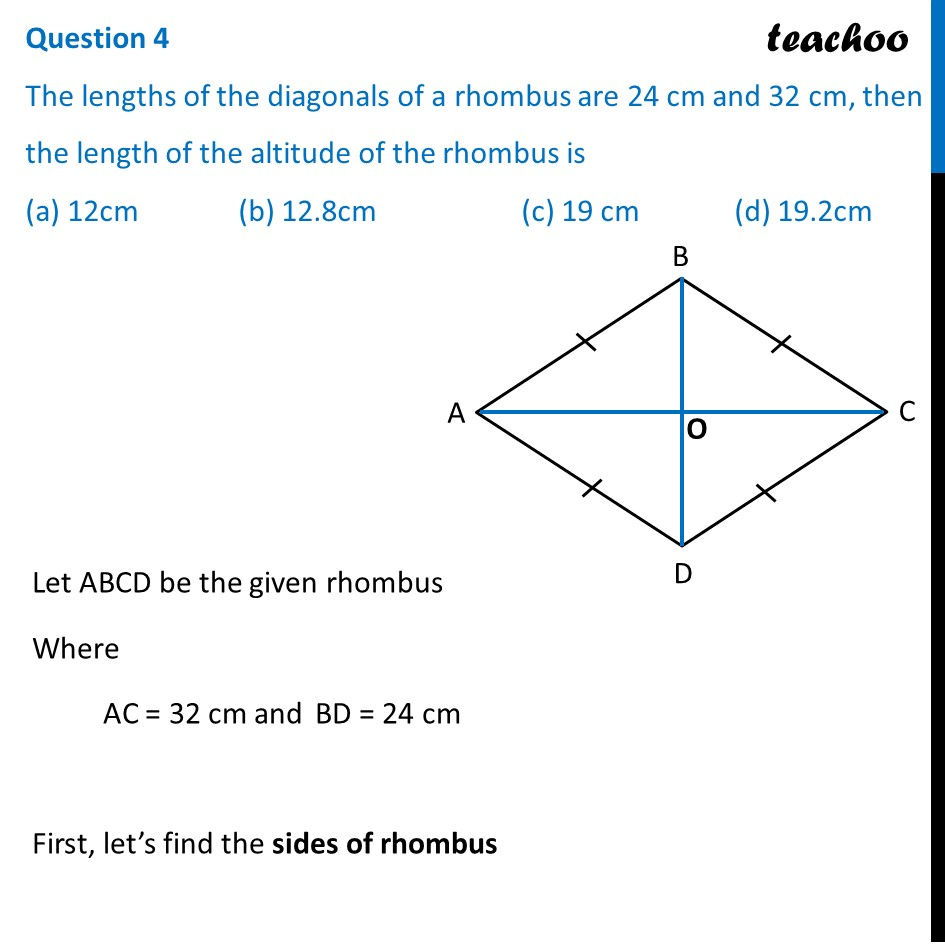googletag.cmd.push(function() { googletag.display('div-gpt-ad-1669298377854-0'); });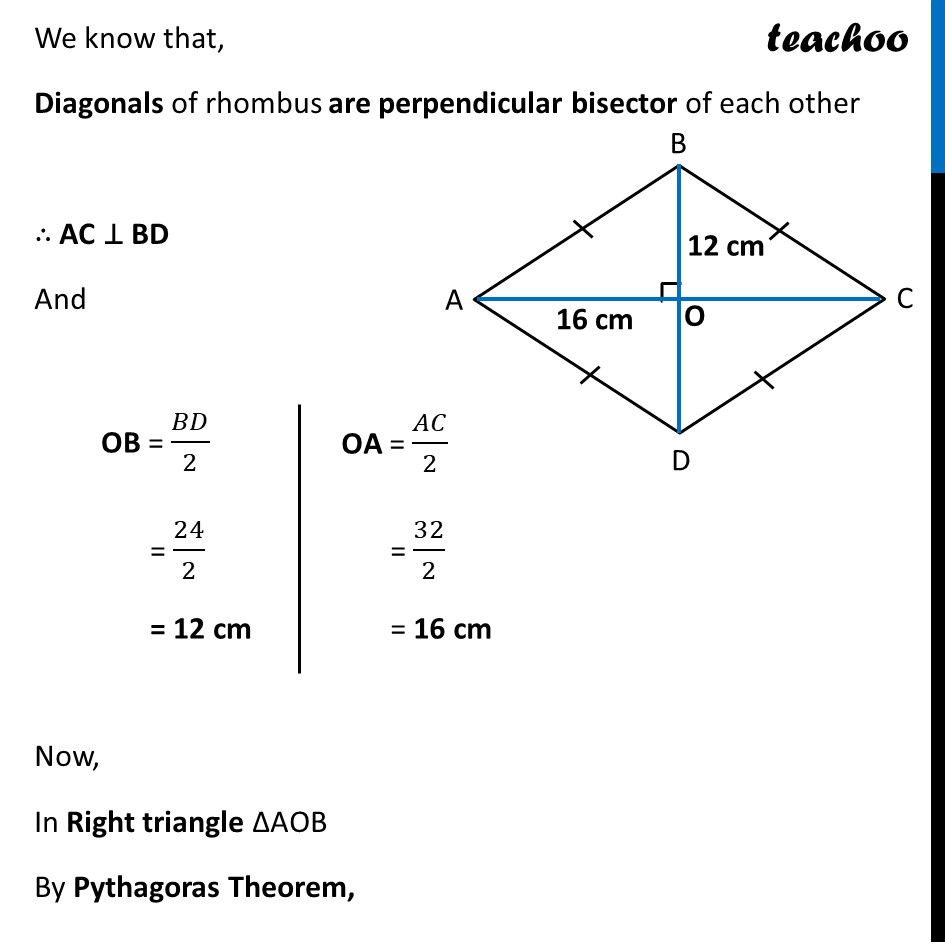(adsbygoogle = window.adsbygoogle || []).push({});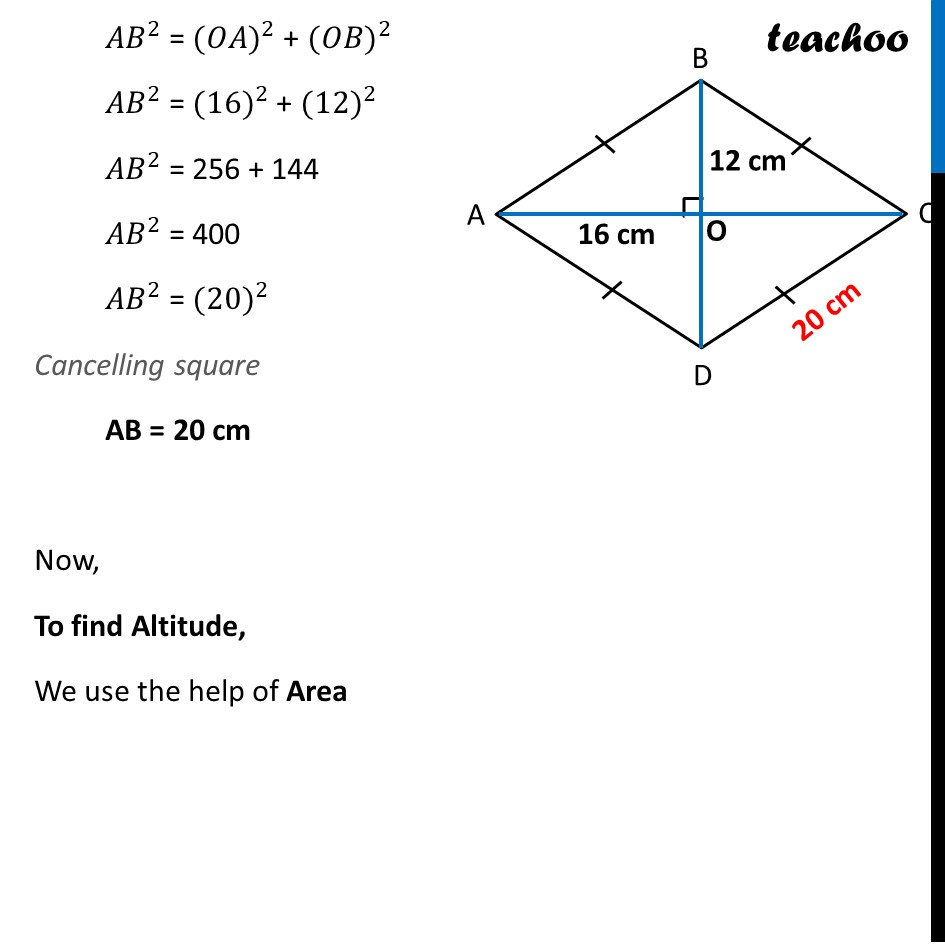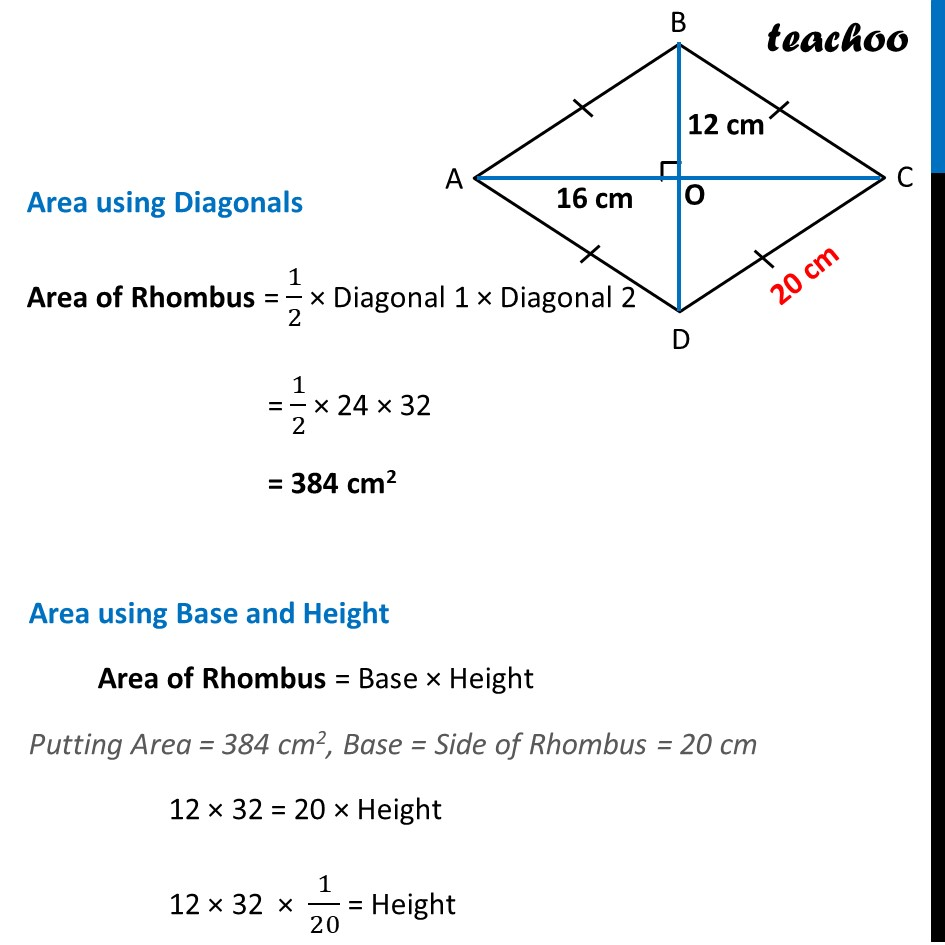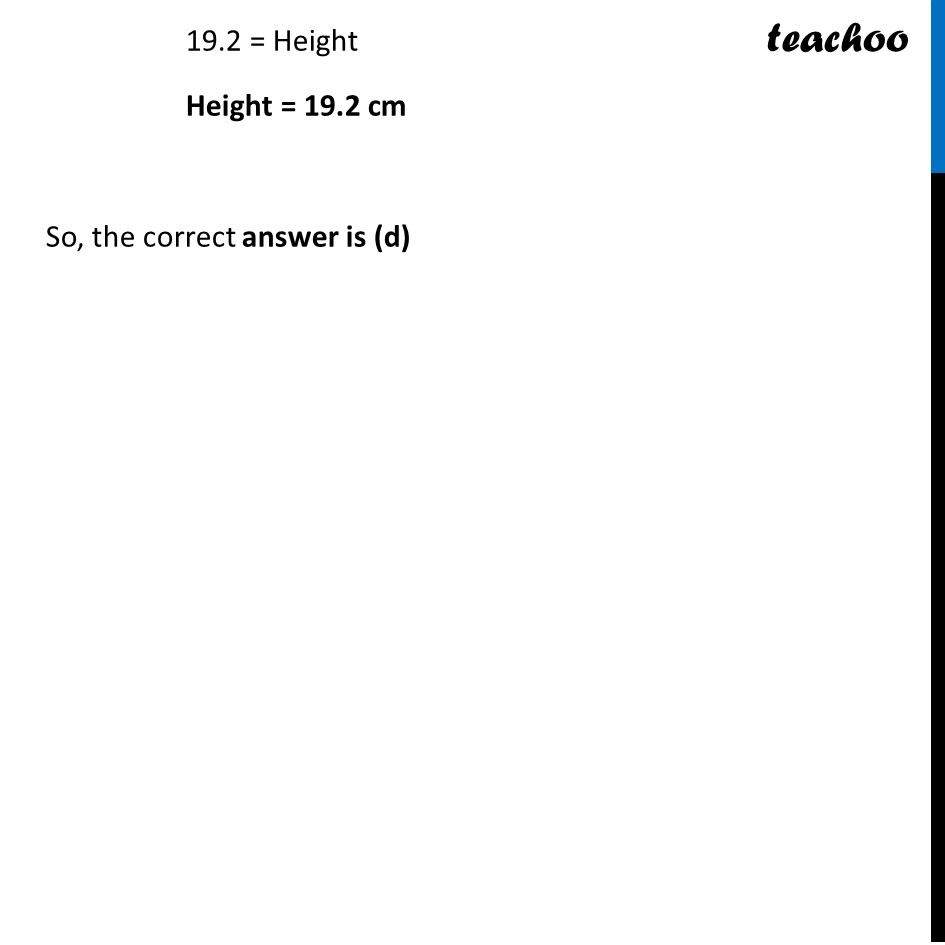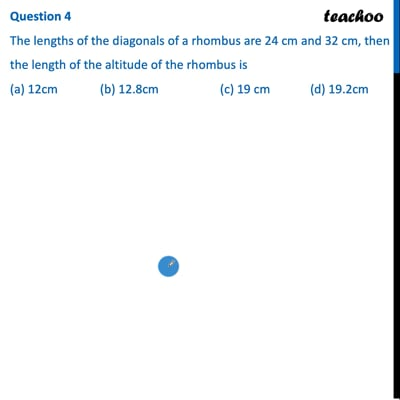This video is only available for Teachoo black users

Learn in your speed, with individual attention - Teachoo Maths 1-on-1 Class

### Transcript

Question 4 The lengths of the diagonals of a rhombus are 24 cm and 32 cm, then the length of the altitude of the rhombus is (a) 12cm (b) 12.8cm (c) 19 cm (d) 19.2cm Let ABCD be the given rhombus Where AC = 32 cm and BD = 24 cm First, let’s find the sides of rhombus We know that, Diagonals of rhombus are perpendicular bisector of each other ∴ AC ⊥ BD And OB = 𝐵𝐷/2 = 24/2 = 12 cm OA = 𝐴𝐶/2 = 32/2 = 16 cm Now, In Right triangle ∆AOB By Pythagoras Theorem, 〖𝐴𝐵〗^2 = 〖(𝑂𝐴)〗^2 + 〖(𝑂𝐵)〗^2 〖𝐴𝐵〗^2 = 〖(16)〗^2 + 〖(12)〗^2 〖𝐴𝐵〗^2 = 256 + 144 〖𝐴𝐵〗^2 = 400 〖𝐴𝐵〗^2 = 〖(20)〗^2 Cancelling square AB = 20 cm Now, To find Altitude, We use the help of Area Area using Diagonals Area of Rhombus = 1/2 × Diagonal 1 × Diagonal 2 = 1/2 × 24 × 32 = 384 cm2 Area using Base and Height Area of Rhombus = Base × Height Putting Area = 384 cm2, Base = Side of Rhombus = 20 cm 12 × 32 = 20 × Height 12 × 32 × 1/20 = Height 19.2 = Height Height = 19.2 cm So, the correct answer is (d)